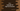# C++ log10() function explanation with examples## Introduction :

The_ log10_ function is used to find out the base 10 logarithms of a number. It has different overloaded methods to take different types of arguments. In this tutorial, we will learn how to use log10() function in C++.

log10 is defined in cmath and it should be included in the program that uses log10.

### Definition as per C++11 :

As per C++11, log10 function is defined as below :

``````double log10 (double x);
float log10 (float x);
long double log10 (long double x);
double log10 (T x);
``````

The last overload is for integral types.

### Parameters :

log10 can take double, float, long double or integral type parameters.

### Return types :

It returns the base 10 logarithms of a number. If the argument is zero or negative, it will raise one pole error or domain error. So, it is a best practice always to verify that the argument is greater than zero.

### Example C++ program :

``````#include <iostream>
#include <cmath>
using namespace std;

int main()
{
double a = 1.43;
float b = 10.03;
long double c = 100.234;
int d = 100;
int e = -13;
int f = 0;

cout << "log10(1.43) : " << log10(a) << endl;
cout << "log10(10.03) : " << log10(b) << endl;
cout << "log10(100.234) : " << log10(c) << endl;
cout << "log10(100) : " << log10(d) << endl;
cout << "log10(-13) : " << log10(e) << endl;
cout << "log10(0) : " << log10(f) << endl;

cout << "log10(1) : " << log10(1) << endl;

return 0;
}
``````We are using different types of arguments in this example. It will print the below output :

``````log10(1.43) : 0.155336
log10(10.03) : 1.0013
log10(100.234) : 2.00102
log10(100) : 2
log10(-13) : nan
log10(0) : -inf
log10(1) : 0
``````

As you can see that for 0 and -13 the result is -inf and nan. If you are using this function in a production application, always make sure to check the argument before using it.